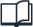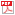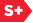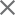Sample Pages### Spie Press Book

Field Guide to Probability, Random Processes, and Random Data Analysis
Format Member Price Non-Member Price

Book Description

Mathematical theory developed in basic courses in engineering and science usually involves deterministic phenomena, and such is the case in solving a differential equation that describes some linear system where both the input and output are deterministic quantities. In practice, however, the input to a linear system, like an imaging system or radar system, may contain a "random" quantity that yields uncertainty about the output. Such systems must be treated by probabilistic methods rather than deterministic methods. For this reason, probability theory and random process theory have become indispensable tools in the mathematical analysis of these kinds of engineering systems. Topics included in this Field Guide are basic probability theory, random processes, random fields, and random data analysis.

Book Details

Date Published: 13 March 2012
Pages: 108
ISBN: 9780819487018
Volume: FG22
Errata

Preface
Glossary of Symbols and Notation
Probability: One Random Variable
Terms and Axioms
Random Variables and Cumulative Distribution
Probability Density Function
Expected Value: Moments
Example: Expected Value
Expected Value: Characteristic Function
Gaussian or Normal Distribution
Other Examples of PDFs: Continuous r.v.
Other Examples of PDFs: Discrete r.v.
Chebyshev Inequality
Law of Large Numbers
Functions of One Random Variable
Example: Square-Law Device
Example: Half-Wave Rectifier
Conditional Probabilities
Conditional Probability: Independent Events
Conditional CDF and PDF
Expected Values
Example: Conditional Expected Value
Probability: Two Random Variables
Joint and Marginal Cumulative Distributions
Joint and Marginal Density Functions
Conditional Distributions and Density Functions
Example: Conditional PDF
Principle of Maximum Likelihood
Independent Random Variables
Expected Value: Moments
Example: Expected Value
Bivariate Gaussian Distribution
Example: Rician Distribution
Functions of Two Random Variables
Sum of Two Random Variables
Product and Quotient of Two Random Variables
Conditional Expectations and Mean-Square Estimation
Sums of N Complex Random Variables
Central Limit Theorem
Central Limit Theorem Example
Phases Uniformly Distributed on (-π, π)
Phases Not Uniformly Distributed on (-π, π)
Example: Phases Uniformly Distributed on (-α, α)
Central Limit Theorem Does Not Apply
Example: Non-Gaussian Limit
Random Processes
Random Processes Terminology
First- and Second-Order Statistics
Stationary Random Processes
Autocorrelation and Autocovariance Functions
Wide-Sense Stationary Process
Example: Correlation and PDF
Time Averages and Ergodicity
Structure Functions
Cross-Correlation and Cross-Covariance Functions
Power Spectral Density (PSD)
Example: Power Spectral Density
PSD Estimation
Bivariate Gaussian Processes
Multivariate Gaussian Processes
Examples of Covariance Function and PSD
Interpretations of Statistical Averages
Random Fields
Random Fields Terminology
Mean and Spatial Covariance Functions
1-D and 3-D Spatial Power Spectrums
2-D Spatial Power Spectrum
Structure Functions
Example: Power Spectral Density
Transformations of Random Processes
Memoryless Nonlinear Transformations
Linear Systems
Expected Values of a Linear System
Example: White Noise
Detection Devices
Zero-Crossing Problem
Random Data Analysis
Tests for Stationarity, Periodicity, and Normality
Nonstationary Data Analysis for Mean
Analysis for Single Time Record
Runs Test for Stationarity
Bibliography
Index